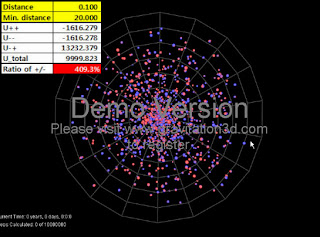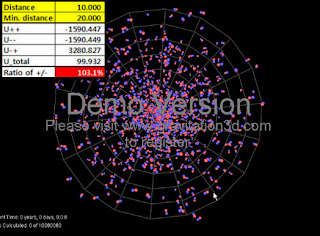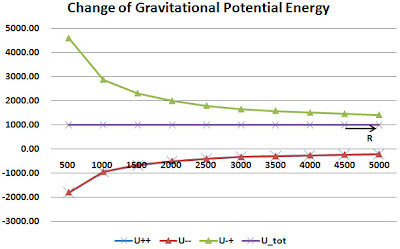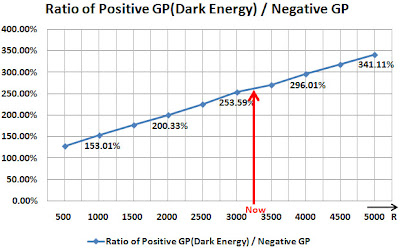# Thread: Important Report on the Dark Energy and Gravitational Potential Energy

1. Hello
I’m sorry. I can’t English well. My native language is not English.

Important Report on the Dark Energy and Gravitational Potential Energy

[Abstract]

Gravity is the force conquering the structure of the universe. By recognizing the components of the universe, we are estimating the quantity of components composing the universe through size of gravity and gravitational potential energy (GPE).

In this paper, it is being shown that the universe can be created and expanded through pair creation of positive energy (mass) and negative energy (mass) from zero energy condition.

Also, GPE is composed by 3 units of,, andwhen negative and positive energy exists,(GPE between negative mass and positive mass) has positive values and is the component that makes repulsive gravitational effect.corresponds with the inner energy of the system and can be interpreted as dark energy.

Also, situations in whichhas much higher value thandepending on the distribution of negative mass and positive mass is possible.

This doesn't mean that 72.1% of dark energy independently exists, but means that explanation from GPE occurring from 4.6%(or 27.9%) of negative energy, which is the same as 4.6%(or include dark matter 27.9%) of positive energy, is possible. Moreover, 4.6%(or 27.9%) of negative energy is the energy which is inevitably required from zero energy, which is the most natural total energy value in the universe.

This discovery implies that our belief that size of gravity and size of components of the universe would always 1:1 correspond was wrong.

I. Introduction
Gravity is the force conquering the structure of the universe. By recognizing the components of the universe, we are estimating the quantity of components composing the universe through size of gravity and GPE.

In estimating some kind of unknown energy quantity of universe components, gravity has been a crucial part. For example, if the universe is composed of some materials and these materials are always moving in equal velocity, unknown repulsive energy to offset this attraction was needed and it was assumed that the size of this energy is the same as the size of the energy of materials and the direction should be the opposite.

From the observation on accelerating expansion of the universe in 1998, we assumed that a repulsive energy bigger than the total energy of visible matter need to exist and called this dark energy. Also, this dark energy has been accepted as an independent energy different from matter or dark matter

We have trusted the above analysis on gravity and GPE had clear validity.
However, through current numerical computation, we found that there was possibility of severe errors in this analysis through gravitational effect.

Negative mass is stable at the state of high energy. So the “problem of the transition of the energy level of minus infinity” does not occur, and thus positive mass and negative mass can exist in the same space-time

In this paper, we show that the universe led to the current structure of the universe, if we assume pair creation of positive energy and negative energy in the early universe.
We looked for size of GPE when negative and positive energy (mass) both exist and try to explain this GPE value regarding to the current size of dark energy.

II. Birth of the universe from zero energy state
Birth of the Universe from Zero Energy State - Bigbang, negative energy,negative mass - YouTube

This is a computer simulation that shows you that the universe led to the current structure of the universe with pair creation of positive energy and negative energy from the zero energy state.

III. Significant characteristics of dark energy and gravitational potential energy
A. Simulation background and method
1) Physical background
a)Initial energy value of the universe
It looks more natural when an initial energy value of universe is 0. Therefore, negative energy is needed to offset positive energy of matters.

If there was pair creation of positive and negative energy in the beginning of the universe, it is estimated that the total value of all positive energy will totally offset the total energy of all negative energy. If we consider only rest mass energy and GPE, the relation below will be valid.b)The size ofandcan be a different during pair creation but this paper has purpose in showing possibility of various GPE values depending on the placement of particles which are pair produced, so the case in whichis equal towill be looked into.

c) Gravitational Potential Energy (GPE)
If negative mass and positive mass coexist, GPE consists of the below three items.GPE between positive masses are negative value.GPE between negative masses are negative value.GPE between positive mass and negative mass are positive value.When the number of negative mass is, and the number of positive mass is, total potential energy is given as follows.2.

3. For example, two pairs exist.Gravitational potential shows significant characteristic when negative mass and positive mass both exist. Whilepositive gravitational potential is produced above,negative gravitational potential are produced. Therefore, total GPE can have various values.

d)Vacuum Energy
Vacuum energy value which is currently known is an energy value that is too big. If this vacuum energy exists, it is difficult to explain why it isn't easily found around us.
In the hypothesis of the pair creation of negative mass and positive mass, Vacuum energy will become exactly 0 because vacuum is the space in which pair creation and pair annihilation of positive and negative energy occurs.

e)Characteristics of the negative mass
For characteristics of the negative mass, refer to below video.
Negative mass, Dark matter, Dark Energy, Bullet Cluster, Antigravity-1 - YouTube

2)Simulation Program
To look into the characteristic of GPE, we used the simulation program named Gravitation3D made by Roice Nelson.

3)Simulation setting
a)Definition of parameter
A few parameters were needed to be defined for simulation.
Distance between pair creation negative energy and positive energy(distance of 1 pair) :Minimum distance between particle pairs for density modification during pair creation :Radius of pair creation range :=500
Particle number of pair creation :=2000ea (1000 pair)

b)Finding mean value
Through Gravitation3D program, 1000 particle pairs (total 2,000ea particles) were produced by random and one mean value (GPE) of each distance value was found 5 times each.

c)Verification on program
To check if the calculated results of the program were correct, we calculated the GPE when 1, 2, and 3 pairs (consist of 15ea potentials) of particles existed by hand and confirmed that this value corresponded to the calculated results of the program.

B. Results of simulation

Important Report for Dark Energy and gravity negative mass negative energy dark matter - YouTube

1) Distance=0.01It was found out thatvalue having positive value could be much higher than. Thus, even though the size of positive mass and negative mass was equal, it could be known that repulsive GPE could be much higher than attractive GPE.

2) Distance =0.10We will describe that an unknown repulsive energyhigher that attraction of visible matter exists for the energy value of above.

3) Distance =0.19According to the observance result of WMAP, it is predicted that current dark energy, dark matter, and matter is approximately 72.1%, 23.3%, and 4.6%, respectively
.
Dark matter and matter correspond to negative gravitational potential because they have attractive gravitational potential and dark energy correspond to positive gravitational potential because it produces repulsive effect.
Therefore, observation ratio of current universe is 72.1/27.9 = 2.584.
Is shows similar condition to 2.63 which was found above.

If conditions are changes, ratio of negative gravitational potential and positive gravitational potential can have various conditions close to 2.58.

4) Distance =1.00Particle pairs show to overlap (in the process being expressed in visible size) because of distance between negative mass and positive mass composing singular particle pairs are relatively close. Overlapping is unrelated to the calculation of GPE.

It can be known that distance between singular particle pairs are much smaller compared to the distance between other particle pairs.
One particle pair corresponds to the cluster of galaxies in the universe structure. From movement characteristic of negative mass and positive mass, galaxies and cluster of galaxies have clustering structure of negative mass in the outside of the galaxy.

5) Distance =10.00C. Accelerating expansion of the universe

Expansion of the universe means increase of the distance between cluster of galaxies or the galaxy while the sizes of individual galaxies are the same.
Structure of galaxies or cluster of galaxies surrounding negative masses in the pair creation model of negative and positive mass is implied.

In this simulation, d0, which is the distance between negative and positive mass, is maintained as a constant in this simulation, or the radius value of the size of the universe increases when d0 decreases.Change of potential energy by change of R radius

Pay attention on the change of negative GPE and positive GPE ratioAs R, which corresponds to the radius of the universe, increases, absolute values of GPE all decrease while the total GPE is mostly maintained.However, ratio of positive gravitational potential and negative gravitational potential increases as the universe expands and this is seen to mean that percentage of repulsive effect (Dark Energy) increases compared to the attractive effect of ordinary matter.

F.Centripetal force effect in the galaxy from dark matter(negative mass) halo out of the galaxy

Centripetal force effect in the galaxy from dark matter(negative mass) halo out of the galaxy - YouTube

If the negative mass is disposed at the outline, the test mass vibrates, and a kind of restoring force (This corresponds to the centripetal force when considering rotation of the galaxy) exists.

This suggests that the halo, dark matter (negative mass) of the external Galaxy could get additional effects of centripetal force to the inner Galaxy.4. G.Observation value of WMAP
1)Pair Creation
Assuming pair creation of negative and positive energy in the beginning of the universe, it is seen that law of conservation of energy should be valid for each pairs for law of conservation of energy to be valid.
According to calculation of the previous paper, it is predicted that size of negative must bigger than size of positive. So2)Some interpretation
According to the observance result of WMAP, it is predicted that current dark energy, dark matter, and matter is approximately 72.1%, 23.3%, and 4.6%, respectively
.
Now, let's correspond to the GPE as follows.
Matter == Negative GP
Dark Matter == Negative GP
Dark Energy == Positive GPIn condition of, results shown above were gained.
This doesn't prove that the size of current negative mass is 2.25 times as positive mass.

However, the above result implies that there is possibility to explain that the size ratio of the 3 predicted energy by using the "hypothesis of pair creation of negative and positive mass."

The ratio above is valid between the 3 physical parameters. Case of this ratio being valid was found through simulation.

H.Results of simulation
1) Even though negative and positive mass have the same size, total GPE can have +, 0, - values depending on the placement of each.

2)value is higher asis smaller and the total GPE also have a high positive value.

3) Looking into the numerical calculation of B)-1)-B)-4), it can be seen that positive gravitational potential valuecan have much higher values than negative gravitational potential values(138% - 3,201%). If we consider the distance of Planck length level, this value can have very high values enough to explain inflation.
From the observation result of WMAP, we know that dark energy value, which generates repulsion, is 2.58 times the total of matter and dark matter. Until now, it is predicted that dark matter produces attraction. Closely look into numbers B)-3).4) Because we judge components of the universe by gravity or GPE, it can be assumed that there is dark energy of 2.63 times or inner energy of 2.63 times regarding the situation ofvalue having approximately 2.63 timesandvalues in number B)-3). There are several situations satisfying 2.58 times.

5) The above results show that 72.1% of dark energy doesn't exist independently, but implies that there is possibility that negative energy having the same size of positive energy can emerge.

6) This "increase of dark energy" doesn't come from a newly generated space, but shows feature of GPE which is made by negative mass and positive mass.

IV. Conclusion
From the simulation result of chapter II and accelerating expansion of the universe, there is possibility that our universe is composed of negative and positive energy (mass). From analysis results of chapter III, total GPE has 3 components and it is known that the total of this GPE can have positive and negative values.

No one has seen dark energy or dark matter. We must remember that we only recognize it through gravitational effect.

Because we judge components of the universe by GPE and gravity which is the slope of GPE, it is estimated that some unknown energy generates repulsive gravitational effect which is 2.63 times higher than the total quantity of matter and dark matter.

This discovery shows possibility that content estimating dark energy quantity through gravitational effect we found can be different from the real fact. This discovery implies that our belief that size of gravity and size of components of the universe would always 1:1 correspond was wrong.

Therefore, it is necessary to try to calculate and observe negative mass more strictly, laying aside the abstract aversion of negative mass.

--- Icarus25. Paper : Important Report on the Dark Energy and Gravitational Potential Energy

http://vixra.org/abs/1110.0019

---Icarus26. A whole new meaning of the word "important".7. What is this "negative Mass " of which you speak? So far as I know there is only one kind of mass. Also there is only one kind of gravity. Your math is complicatied but it seems to be counting things that don't exist. What happens to your math if you replace all mention of negative mass or negative gravity with the value "0"?Bookmarks
 Posting Permissions
 You may not post new threads You may not post replies You may not post attachments You may not edit your posts   BB code is On Smilies are On [IMG] code is On [VIDEO] code is On HTML code is Off Trackbacks are Off Pingbacks are Off Refbacks are On Terms of Use Agreement